Worksheets and No Prep Teaching Resources Math Worksheets Shapes Geometry Printables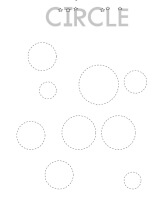Circles Worksheets, Printables, and Lesson Plans

Kindergarten Geometry - Introduction to Drawing Some Simple Polygons

Shapes

Kindergarten Geometry: Tracing, Drawing, and Counting Shapes
CirclesTrace Circles (LARGE Circles - few per page) Trace and Count Circles (LARGE Circles - few per page) Trace Circles (a little smaller - more circles per page) Trace and Count Circles (a little smaller - more circles per page) Trace Circles (very small circles) Trace and Count Circles (very small circles)

Rectangles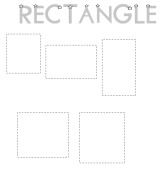Trace Rectangles (LARGE Rectangles - few per page) Trace and Count Rectangles (LARGE Rectangles - few per page) Trace Rectangles (a little smaller) Trace and Count Rectangles (a little smaller) Trace Rectangles (very small) Trace and Count Rectangles (very small)

Squares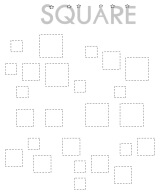Trace Squares (LARGE Squares - few per page) Trace and Count Squares (LARGE Squares - few per page) Trace Squares (a little smaller) Trace and Count Squares (a little smaller) Trace Squares (very small) Trace and Count Squares (very small)

Triangles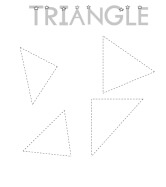Trace Triangles (LARGE Triangles - few per page) Trace and Count Triangles (LARGE Triangles - few per page) Trace Triangles (a little smaller) Trace and Count Triangles (a little smaller) Trace Triangles (very small) Trace and Count Triangles (very small)

Circles, Squares, Rectangles, and Triangles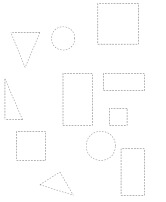Trace Circles, Squares, Rectangles, and Triangles (LARGE) Trace and Count Circles, Squares, Rectangles, and Triangles (LARGE) Trace Circles, Squares, Rectangles, and Triangles (a little smaller) Trace and Count Circles, Squares, Rectangles, and Triangles (a little smaller) Trace Circles, Squares, Rectangles, and Triangles (very small) Trace and Count Circles, Squares, Rectangles, and Triangles (very small)

Kindergarten Geometry: Understanding Shape Words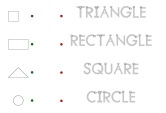Match Shapes with Spelling of Word

Kindergarten Geometry: Tracing and then Copying a Shape
Larger Shapes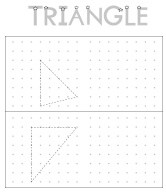Circles: Trace and Copy Circles Rectangles: Trace and Copy Rectangles Squares: Trace and Copy Squares Triangles: Trace and Copy Triangles

Smaller Shapes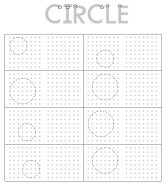Circles: Trace and Copy Circles Rectangles: Trace and Copy Rectangles Squares: Trace and Copy Squares Triangles: Trace and Copy Triangles

Shapes - Counting Number of Sides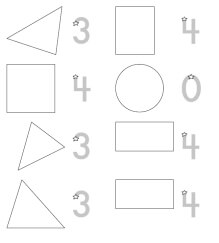Shapes - Counting Number of Sides (traceable answer given for each)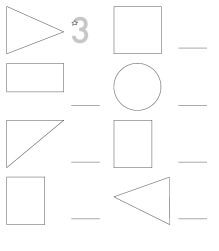Shapes - Counting Number of Sides (traceable answer for first shape only)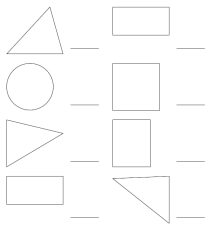Shapes - Counting Number of Sides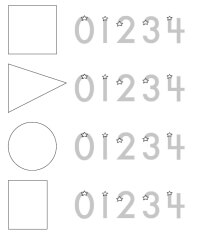Shapes - Counting Number of Sides - Circle the Answer

Draw Shapes and Trace Word - Circle, Rectangle, Square, Triangle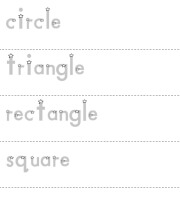Draw Shapes and Trace Word (uppercase words) Draw Shapes and Trace Word (lowercase words)

Complete Shapes - Circle, Rectangle, Square, Triangle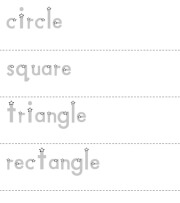Complete the Shapes

Shape Mazes

Following Directions

Shapes Math Skills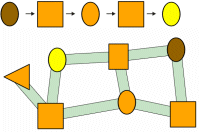Number Mazes and Shapes Mazes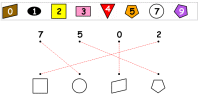Matching Shapes, Numbers, and Colors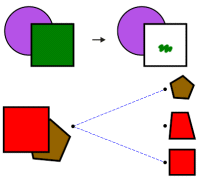Combined Shapes: Coloring and Matching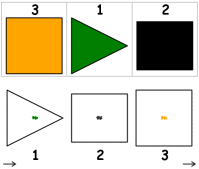Ordering Shapes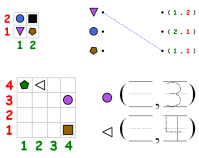Grids: Shapes, Positions, Writing Numbers

Shapes - Visual Skills
Visual Discrimination: Matching Shapes
Match shapes

Visual Closure
Incomplete Pictures
Match shapes (easier)
Match shapes (more difficult - more lines are missing)

Visual Spatial Relationships
Draw one shape
Draw 2-3 shapes
Draw shapes plus coloring
(one row) Select the correct statement
(one row) Select the statement that is wrong
(two rows) Select the correct statement
(two rows) Select the statement that is wrong

Visual Figure-Ground
Color the shapes

Complete the Square (pick the missing shape)
Easier Shapes
Pick the missing shape: Choices are not rotated
Pick the missing shape: Choices are rotated
Pick the missing shape: Choices may be rotated
More Difficult Shapes
Pick the missing shape: Choices are not rotated
Pick the missing shape: Choices are rotated
Pick the missing shape: Choices may be rotated

Complete the Shape

Redraw, Enlarge, and Reduce Shapes

Puzzles

Have a suggestion or would like to leave feedback?